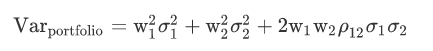# Correlation Coefficient and Variance

Which one of the following statements about correlation is NOT correct?

A)

Potential benefits from diversification arise when correlation is less than +1.

B)

If the correlation coefficient were -1, a zero variance portfolio could be constructed.

C)

If the correlation coefficient were 0, a zero variance portfolio could be constructed.

I had to think this one through, is this logic correct? Since the formula for Variance isand we want 0 variance, that would mean we need the third term 2w1*w2*Corr1,2*stdev1*stdev2 to equal negative some number. That way we can add the other two positive terms to maybe come up with zero variance?

You’re ready to take on the CFA® Program, so stop guessing where you should begin. You give us your study dates, we’ll give you the study plan. Our adaptive activity feed breaks down your 300 hours into bite-sized weekly tasks that fit into your life.

The correct answer is B, I forgot to mention above.

wichertj wrote:“Mmmmmm, something…” - H. Simpson

wichertj wrote:
I had to think this one through, is this logic correct? Since the formula for Variance isand we want 0 variance, that would mean we need the third term 2w1*w2*Corr1,2*stdev1*stdev2 to equal negative some number. That way we can add the other two positive terms to maybe come up with zero variance?

It is.

Simplify the complicated side; don't complify the simplicated side.

Financial Exam Help 123: The place to get help for the CFA® exams
http://financialexamhelp123.com/

The situation becomes even simpler for c, since the third term becomes 0.  The individual asset variances are >=0 and w1+w2=1 with w1,w2>=0.  About the only way I can think of to get portfolio variance = 0 is to put 100% weight on an asset with variance = 0.

“Mmmmmm, something…” - H. Simpson

Thanks for clearing it up! It makes sense now.

I’m too tired to crank the math, but if I remember correctly, with a perfect negative correlation, you get a zero-variance portfolio if w1/w2 = sd2/sd1 (ie if you choose weights such that the ratio of the weights is the inverse of the ratios of the standard deviations). In other words if you had standard deviations of 10% for asset 1 and 5% for asset two, weights of 1/3 for asset 1 and 2/3 for asset 2 would give a zero-variance portfolio.

You keep using that word.  I do not think it means what you think it means.